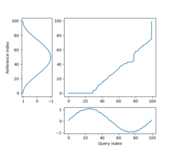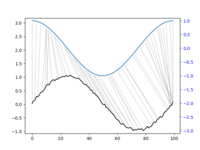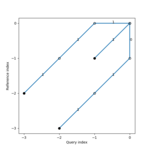# dtw-python: Dynamic Time Warping in Python

The dtw-python module is a faithful Python equivalent of the R package; it provides the same algorithms and options.

Warning

The (pip) package name is `dtw-python`; the import statement is just `import dtw`.

## Installation

To install the stable version of the package, issue the following command:

``````pip install dtw-python
``````

## Getting started

Begin from the installed documentation:

``````> from dtw import *
> ?dtw
> help(DTW)
``````

Note

Note: the documentation for the Python module is auto-generated from the R version. It may contain minor inconsistencies.

## Online documentation

The package documentation can also be browsed online.

## Quickstart

``````import numpy as np

## A noisy sine wave as query
idx = np.linspace(0,6.28,num=100)
query = np.sin(idx) + np.random.uniform(size=100)/10.0

## A cosine is for template; sin and cos are offset by 25 samples
template = np.cos(idx)

## Find the best match with the canonical recursion formula
from dtw import *
alignment = dtw(query, template, keep_internals=True)

## Display the warping curve, i.e. the alignment curve
alignment.plot(type="threeway")

## Align and plot with the Rabiner-Juang type VI-c unsmoothed recursion
dtw(query, template, keep_internals=True,
step_pattern=rabinerJuangStepPattern(6, "c"))\
.plot(type="twoway",offset=-2)

## See the recursion relation, as formula and diagram
print(rabinerJuangStepPattern(6,"c"))
rabinerJuangStepPattern(6,"c").plot()

## And much more!
``````Try online!

## Differences with R

### Indices are 0-based

R uses 1-based indexing, whereas Python uses 0-based arrays. Wherever indices are returned (most notably in the `.index1`, `.index2`, `.index1s` and `.index2s` attributes of alignments), these must be assumed to be 0-based in Python. Hence, indices can be used as subscripts in both environments as expected.

### Object-oriented methods

Python OO method calls use the postfix "dot" notation. This mostly affects the `plot()` methods. Note that non-overloaded functional style such as `dtwPlotThreeWay` are unaffected. Hence:

``````## In R
plot(alignment, type="threeway")

## In Python
alignment.plot(type="threeway")
## or
dtwPlotThreeWay(alignment)
``````

### The alignment class

The class name of alignment objects in `DTW` (all capitals) in Python. Its attributes are accessed with the usual "dot" notation (R uses `\$` as for lists).

### Dots vs underscores

R commonly uses the dot (`.`) separator for function argument names, while Python uses the underscore (`_`) for the same purpose. The function prototypes reflect this difference. Also, Python does not accept abbreviated argument names. Therefore:

``````## In R
alignment = dtw(query, template, keep.int=TRUE)

## In Python
alignment = dtw(query, template, keep_internals=True)
``````

### Plots

The graphing functions have been re-implemented within the `matplotlib` framework. They return `axes` objects, which can be used to customize the plot appearance.